# Tutorials

## Quick Start

### Getting started with DIPY

In diffusion MRI (dMRI) usually we use three types of files, a Nifti file with the diffusion weighted data, and two text files one with b-values and one with the b-vectors.

### Introduction to Basic Tracking

Local fiber tracking is an approach used to model white matter fibers by creating streamlines from local directional information. The idea is as follows: if the local directionality of a tract/pathway segment is known, one can integrate along those directions to build a complete representation of that structure. Local fiber tracking is widely used in the field of diffusion MRI because it is simple and robust.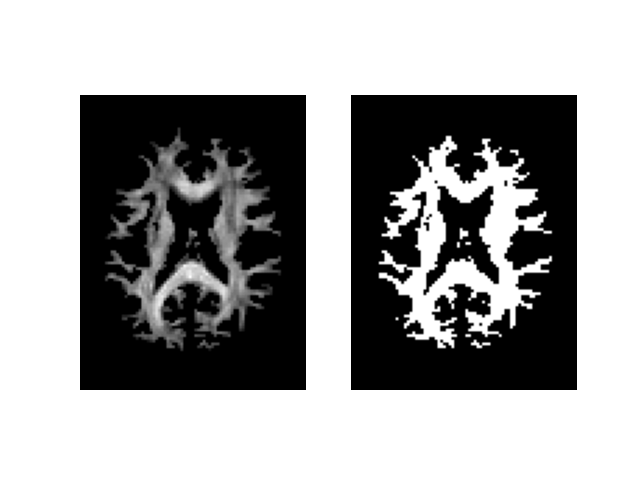## Preprocessing

This example shows how you can create gradient tables and sphere objects using DIPY.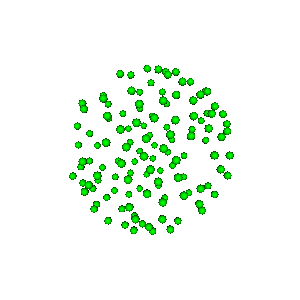### Brain segmentation with median_otsu

We show how to extract brain information and mask from a b0 image using DIPY’s segment.mask module.

### SNR estimation for Diffusion-Weighted Images

Computing the Signal-to-Noise-Ratio (SNR) of DW images is still an open question, as SNR depends on the white matter structure of interest as well as the gradient direction corresponding to each DWI.

### Reslice diffusion datasets

Often in imaging it is common to reslice images in different resolutions. Especially in dMRI we usually want images with isotropic voxel size as they facilitate most tractography algorithms. In this example we show how you can reslice a dMRI dataset to have isotropic voxel size.

### Between-volumes Motion Correction on DWI datasets

During a dMRI acquisition, the subject motion inevitable. This motion implies a misalignment between N volumes on a dMRI dataset. A common way to solve this issue is to perform a registration on each acquired volume to a reference b = 0. [JenkinsonSmith01]

## Denoising

### Patch2Self: Self-Supervised Denoising via Statistical Independence

Patch2Self [Fadnavis20] is a self-supervised learning method for denoising DWI data, which uses the entire volume to learn a full-rank locally linear denoiser for that volume. By taking advantage of the oversampled q-space of DWI data, Patch2Self can separate structure from noise without requiring an explicit model for either.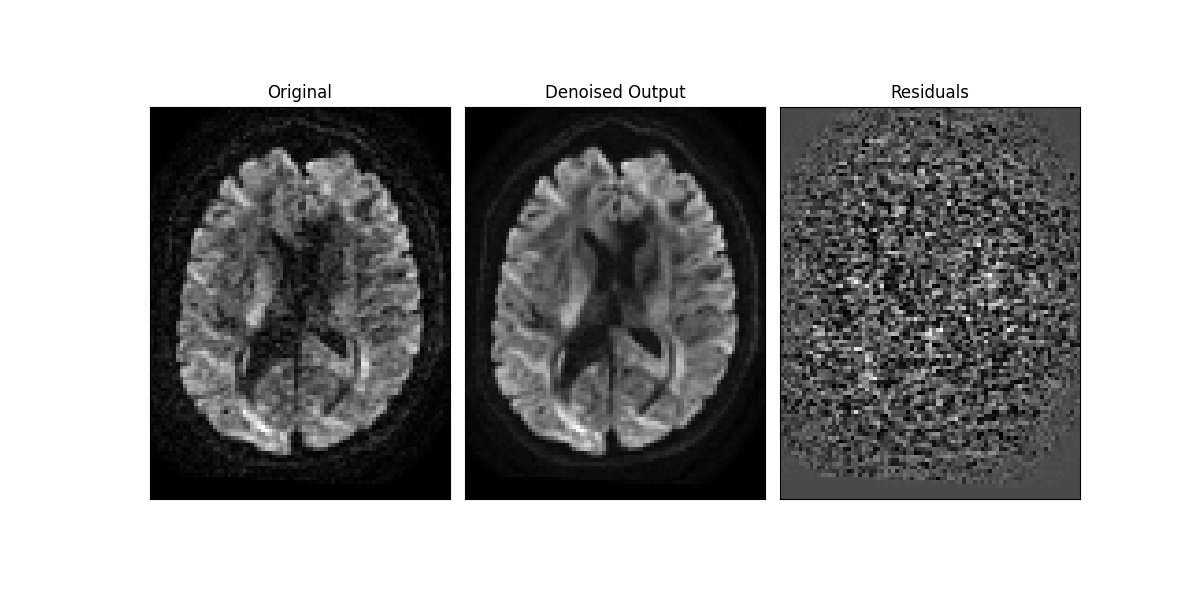### Denoise images using Non-Local Means (NLMEANS)

Using the non-local means filter [Coupe08] and [Coupe11] and you can denoise 3D or 4D images and boost the SNR of your datasets. You can also decide between modeling the noise as Gaussian or Rician (default).

### Denoise images using Local PCA via empirical thresholds

PCA-based denoising algorithms are effective denoising methods because they explore the redundancy of the multi-dimensional information of diffusion-weighted datasets. In this example, we will show how to perform the PCA-based denoising using the algorithm proposed by Manjon et al. [Manjon2013].

### Denoise images using the Marcenko-Pastur PCA algorithm

The PCA-based denoising algorithm exploits the redundancy across the diffusion-weighted images [Manjon2013], [Veraart2016a]. This algorithm has been shown to provide an optimal compromise between noise suppression and loss of anatomical information for different techniques such as DTI [Manjon2013], spherical deconvolution [Veraart2016a] and DKI [Henri2018].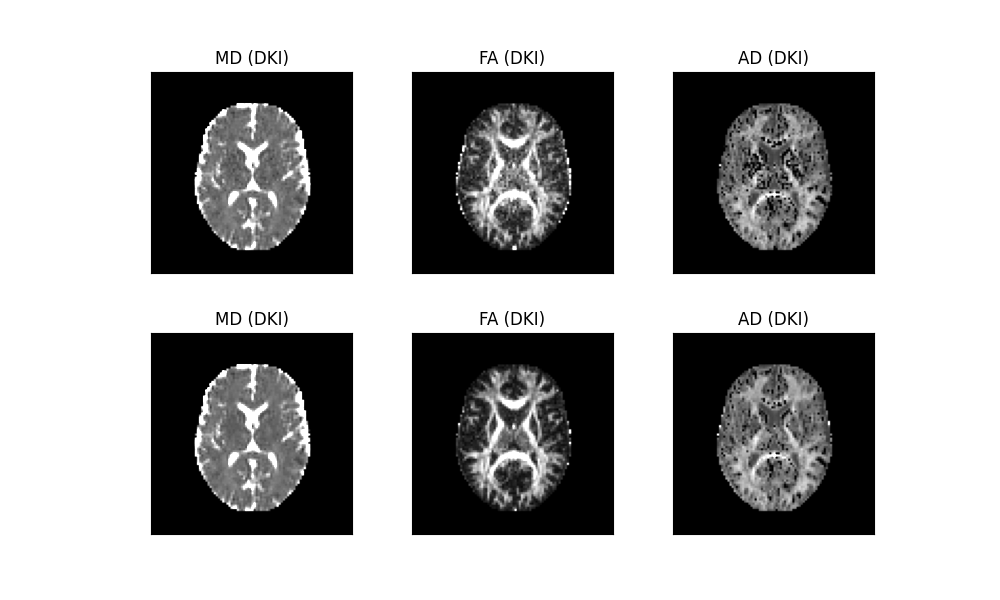### Suppress Gibbs oscillations

Magnetic Resonance (MR) images are reconstructed from the Fourier coefficients of acquired k-space images. Since only a finite number of Fourier coefficients can be acquired in practice, reconstructed MR images can be corrupted by Gibbs artefacts, which is manifested by intensity oscillations adjacent to edges of different tissues types . Although this artefact affects MR images in general, in the context of diffusion-weighted imaging, Gibbs oscillations can be magnified in derived diffusion-based estimates , .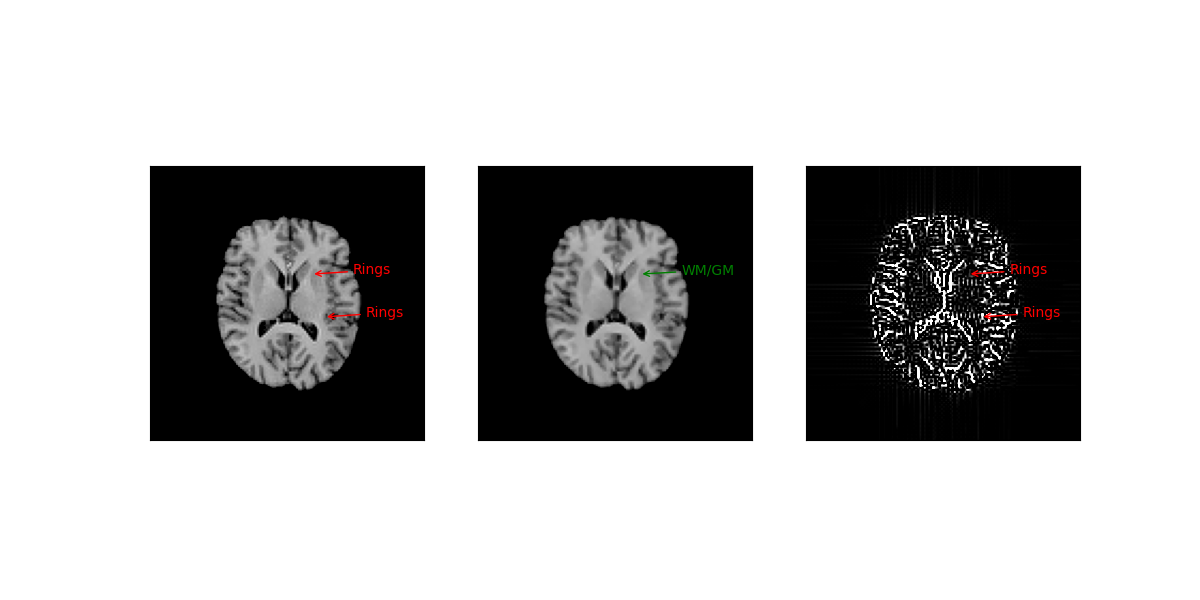## Reconstruction

### Reconstruction with Constrained Spherical Deconvolution

This example shows how to use Constrained Spherical Deconvolution (CSD) introduced by Tournier et al. [Tournier2007].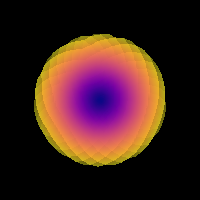### Reconstruction with Multi-Shell Multi-Tissue CSD

This example shows how to use Multi-Shell Multi-Tissue Constrained Spherical Deconvolution (MSMT-CSD) introduced by Tournier et al. [Jeurissen2014]. This tutorial goes through the steps involved in implementing the method.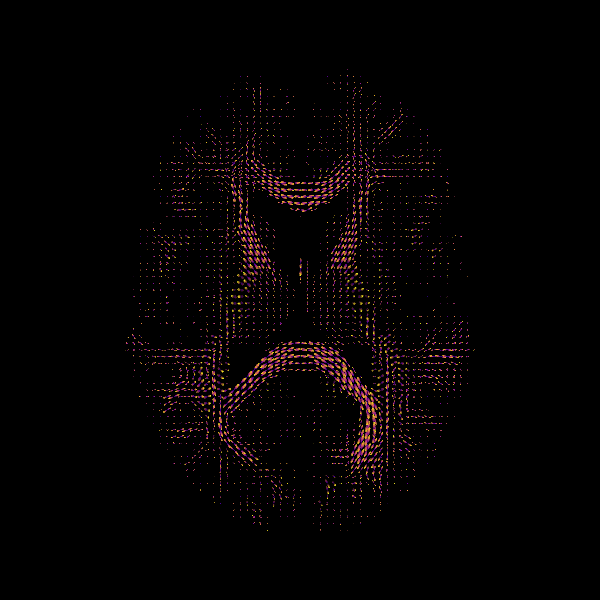### Crossing invariant fiber response function with FORECAST model

We show how to obtain a voxel specific response function in the form of axially symmetric tensor and the fODF using the FORECAST model from [Anderson2005] , [Kaden2016] and [Zucchelli2017].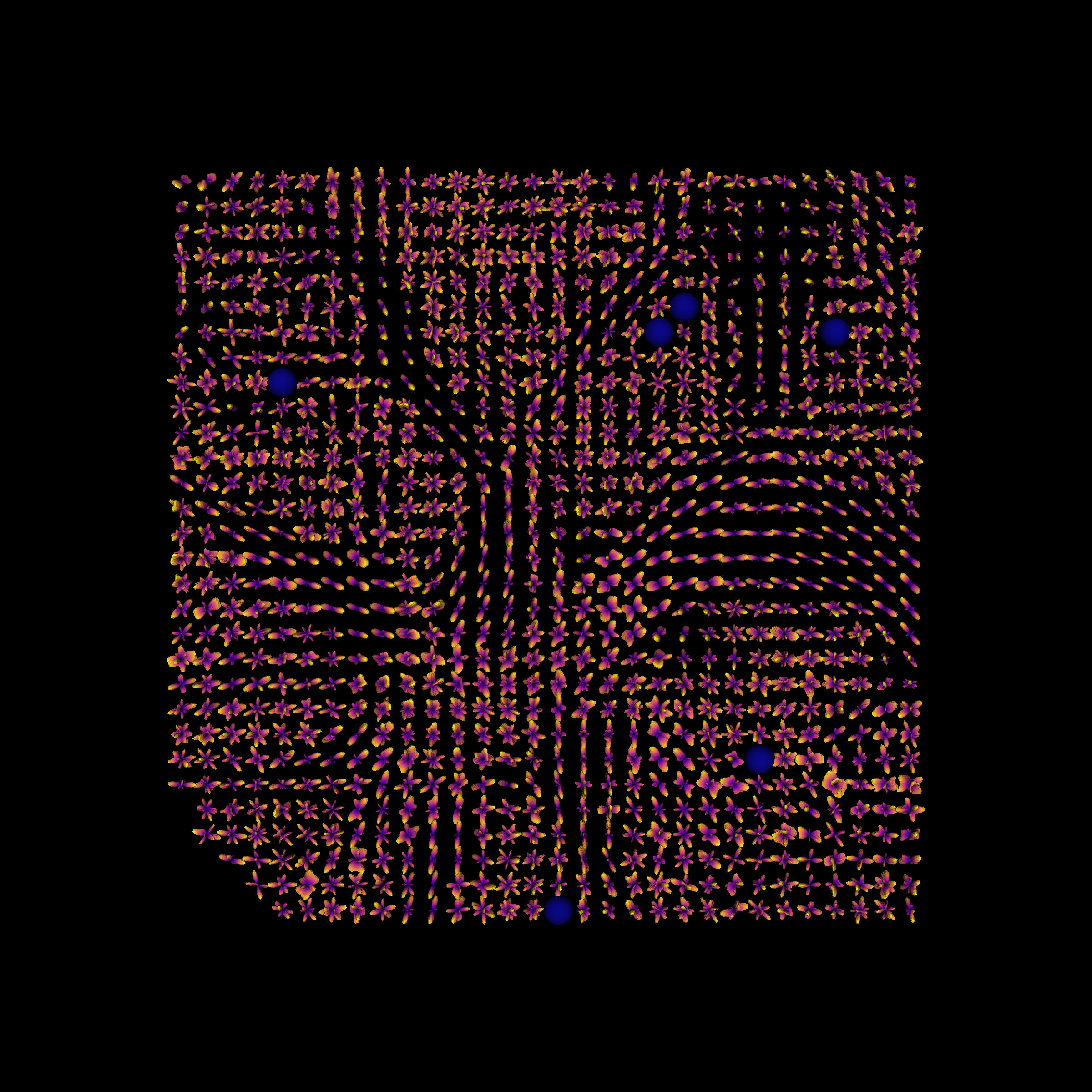### Continuous and analytical diffusion signal modelling with 3D-SHORE

We show how to model the diffusion signal as a linear combination of continuous functions from the SHORE basis [Merlet2013], [Özarslan2008], [Özarslan2009]. We also compute the analytical Orientation Distribution Function (ODF).

### Calculate SHORE scalar maps

We show how to calculate two SHORE-based scalar maps: return to origin probability (RTOP) [Descoteaux2011] and mean square displacement (MSD) [Wu2007], [Wu2008] on your data. SHORE can be used with any multiple b-value dataset like multi-shell or DSI.

### Continuous and analytical diffusion signal modelling with MAP-MRI

We show how to model the diffusion signal as a linear combination of continuous functions from the MAP-MRI basis [Ozarslan2013]. This continuous representation allows for the computation of many properties of both the signal and diffusion propagator.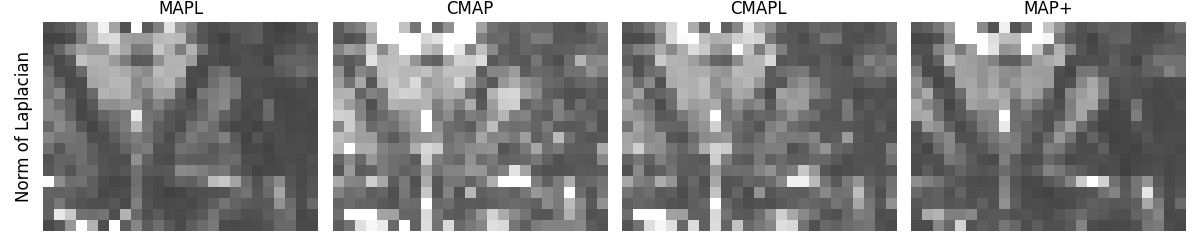### Estimating diffusion time dependent q-space indices using qt-dMRI

Effective representation of the four-dimensional diffusion MRI signal – varying over three-dimensional q-space and diffusion time – is a sought-after and still unsolved challenge in diffusion MRI (dMRI). We propose a functional basis approach that is specifically designed to represent the dMRI signal in this qtau-space [Fick2017]. Following recent terminology, we refer to our qtau-functional basis as $$q\tau$$-dMRI. We use GraphNet regularization – imposing both signal smoothness and sparsity – to drastically reduce the number of diffusion-weighted images (DWIs) that is needed to represent the dMRI signal in the qtau-space. As the main contribution, $$q\tau$$-dMRI provides the framework to – without making biophysical assumptions – represent the $$q\tau$$-space signal and estimate time-dependent q-space indices ($$q\tau$$-indices), providing a new means for studying diffusion in nervous tissue. $$q\tau$$-dMRI is the first of its kind in being specifically designed to provide open interpretation of the $$q\tau$$-diffusion signal.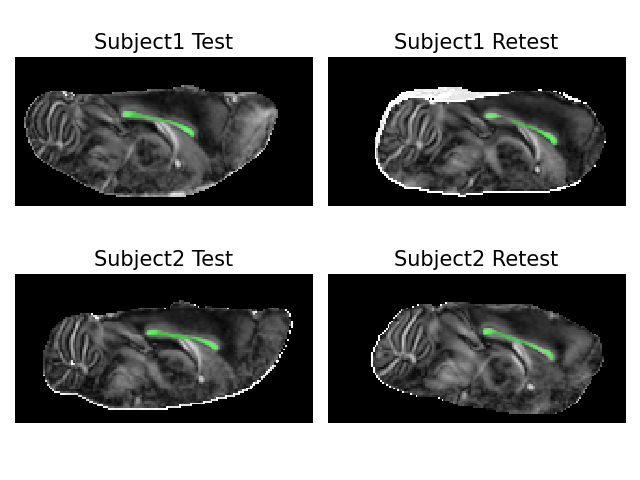### Reconstruction of the diffusion signal with the Tensor model

The diffusion tensor model is a model that describes the diffusion within a voxel. First proposed by Basser and colleagues [Basser1994], it has been very influential in demonstrating the utility of diffusion MRI in characterizing the micro-structure of white matter tissue and of the biophysical properties of tissue, inferred from local diffusion properties and it is still very commonly used.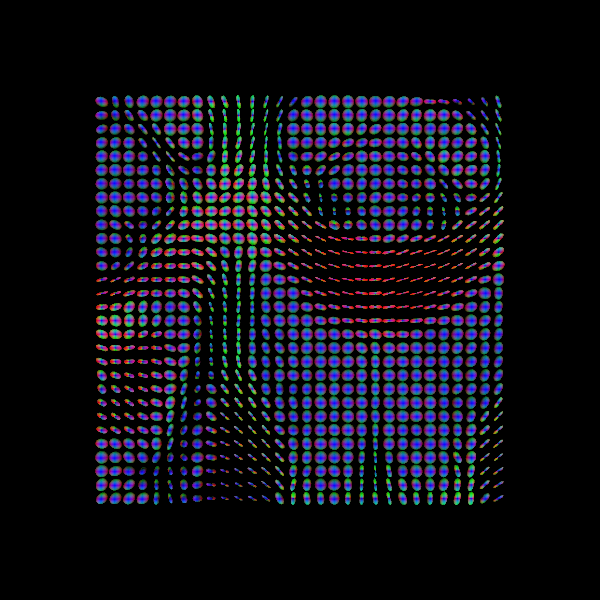### Using the RESTORE algorithm for robust tensor fitting

The diffusion tensor model takes into account certain kinds of noise (thermal), but not other kinds, such as “physiological” noise. For example, if a subject moves during acquisition of one of the diffusion-weighted samples, this might have a substantial effect on the parameters of the tensor fit calculated in all voxels in the brain for that subject. One of the pernicious consequences of this is that it can lead to wrong interpretation of group differences. For example, some groups of participants (e.g. young children, patient groups, etc.) are particularly prone to motion and differences in tensor parameters and derived statistics (such as FA) due to motion would be confounded with actual differences in the physical properties of the white matter. An example of this was shown in a paper by Yendiki et al. [Yendiki2013].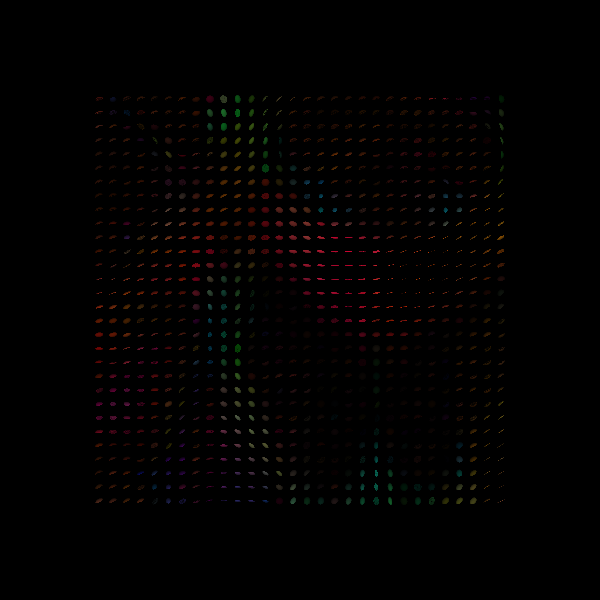### Using the free water elimination model to remove DTI free water contamination

As shown previously (see Reconstruction of the diffusion signal with the Tensor model), the diffusion tensor model is a simple way to characterize diffusion anisotropy. However, in regions near the ventricles and parenchyma, anisotropy can be underestimated by partial volume effects of the cerebral spinal fluid (CSF). This free water contamination can particularly corrupt Diffusion Tensor Imaging analysis of microstructural changes when different groups of subjects show different brain morphology (e.g. brain ventricle enlargement associated with brain tissue atrophy that occurs in several brain pathologies and aging).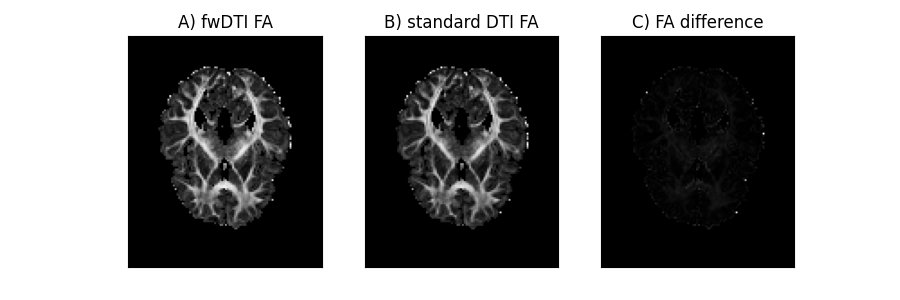### Reconstruction of the diffusion signal with the kurtosis tensor model

The diffusion kurtosis model is an expansion of the diffusion tensor model (see Reconstruction of the diffusion signal with the Tensor model). In addition to the diffusion tensor (DT), the diffusion kurtosis model quantifies the degree to which water diffusion in biological tissues is non-Gaussian using the kurtosis tensor (KT) [Jensen2005].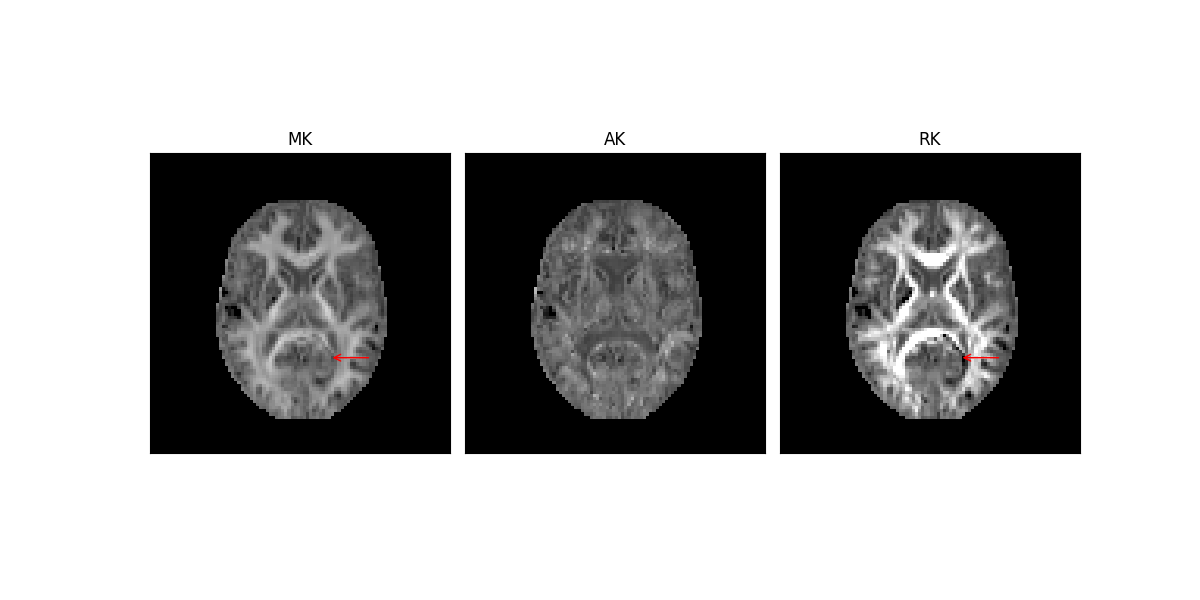### Mean signal diffusion kurtosis imaging (MSDKI)

Diffusion Kurtosis Imaging (DKI) is one of the conventional ways to estimate the degree of non-Gaussian diffusion (see Reconstruction of the diffusion signal with the kurtosis tensor model) [Jensen2005]. However, a limitation of DKI is that its measures are highly sensitive to noise and image artefacts. For instance, due to the low radial diffusivities, standard kurtosis estimates in regions of well-aligned voxel may be corrupted by implausible low or even negative values.

### Reconstruction of the diffusion signal with the WMTI model

DKI can also be used to derive concrete biophysical parameters by applying microstructural models to DT and KT estimated from DKI. For instance, Fieremans et al. [Fierem2011] showed that DKI can be used to estimate the contribution of hindered and restricted diffusion for well-aligned fibers - a model that was later referred to as the white matter tract integrity WMTI technique [Fierem2013]. The two tensors of WMTI can be also interpreted as the influences of intra- and extra-cellular compartments and can be used to estimate the axonal volume fraction and diffusion extra-cellular tortuosity. According to previous studies [Fierem2012] [Fierem2013], these latter measures can be used to distinguish processes of axonal loss from processes of myelin degeneration.

### Reconstruct with Constant Solid Angle (Q-Ball)

We show how to apply a Constant Solid Angle ODF (Q-Ball) model from Aganj et al. [Aganj2010] to your datasets.

### Reconstruct with Diffusion Spectrum Imaging

We show how to apply Diffusion Spectrum Imaging [Wedeen08] to diffusion MRI datasets of Cartesian keyhole diffusion gradients.

### Calculate DSI-based scalar maps

We show how to calculate two DSI-based scalar maps: return to origin probability (RTOP) [Descoteaux2011] and mean square displacement (MSD) [Wu2007], [Wu2008] on your dataset.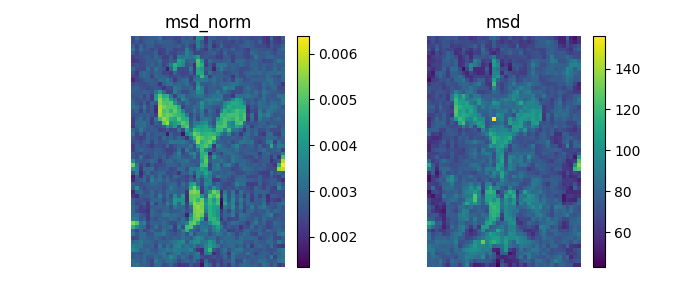### Reconstruct with Generalized Q-Sampling Imaging

We show how to apply Generalized Q-Sampling Imaging [Yeh2010] to diffusion MRI datasets. You can think of GQI as an analytical version of DSI orientation distribution function (ODF) (Garyfallidis, PhD thesis, 2012).

### DSI Deconvolution vs DSI

An alternative method to DSI is the method proposed by [Canales10] which is called DSI with Deconvolution. This algorithm is using Lucy-Richardson deconvolution in the diffusion propagator with the goal to create sharper ODFs with higher angular resolution.

### Reconstruction with the Sparse Fascicle Model

In this example, we will use the Sparse Fascicle Model (SFM) [Rokem2015], to reconstruct the fiber Orientation Distribution Function (fODF) in every voxel.

### Intravoxel incoherent motion

The intravoxel incoherent motion (IVIM) model describes diffusion and perfusion in the signal acquired with a diffusion MRI sequence that contains multiple low b-values. The IVIM model can be understood as an adaptation of the work of Stejskal and Tanner [Stejskal65] in biological tissue, and was proposed by Le Bihan [LeBihan84]. The model assumes two compartments: a slow moving compartment, where particles diffuse in a Brownian fashion as a consequence of thermal energy, and a fast moving compartment (the vascular compartment), where blood moves as a consequence of a pressure gradient. In the first compartment, the diffusion coefficient is $$\mathbf{D}$$ while in the second compartment, a pseudo diffusion term $$\mathbf{D^*}$$ is introduced that describes the displacement of the blood elements in an assumed randomly laid out vascular network, at the macroscopic level. According to [LeBihan84], $$\mathbf{D^*}$$ is greater than $$\mathbf{D}$$.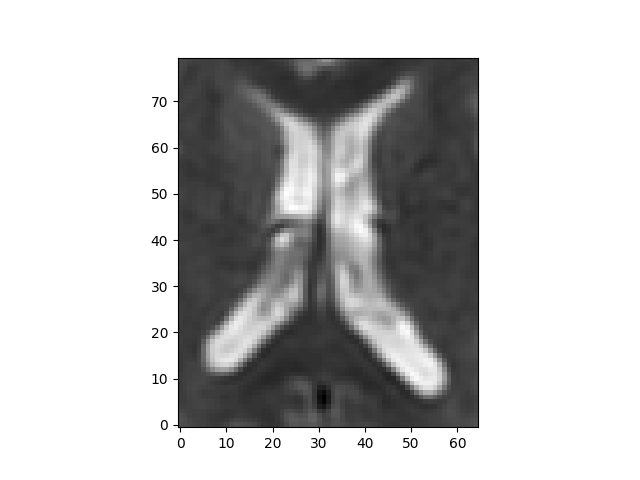### K-fold cross-validation for model comparison

Different models of diffusion MRI can be compared based on their accuracy in fitting the diffusion signal. Here, we demonstrate this by comparing two models: the diffusion tensor model (DTI) and Constrained Spherical Deconvolution (CSD). These models differ from each other substantially. DTI approximates the diffusion pattern as a 3D Gaussian distribution, and has only 6 free parameters. CSD, on the other hand, fits many more parameters. The models aare also not nested, so they cannot be compared using the log-likelihood ratio.

### Signal Reconstruction Using Spherical Harmonics

This example shows how you can use a spherical harmonics (SH) function to reconstruct any spherical function using DIPY. In order to generate a signal, we will need to generate an evenly distributed sphere. Let’s import some standard packages.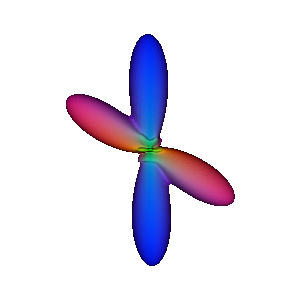### Reconstruct with Q-space Trajectory Imaging (QTI)

Q-space trajectory imaging (QTI) by Westin et al.  is a general framework for analyzing diffusion-weighted MRI data acquired with tensor-valued diffusion encoding. This tutorial briefly summarizes the theory and provides an example of how to estimate the diffusion and covariance tensors using DIPY.

### Applying positivity constraints to Q-space Trajectory Imaging (QTI+)

Q-space trajectory imaging (QTI)  with applied positivity constraints (QTI+) is an estimation framework proposed by Herberthson et al.  which enforces necessary constraints during the estimation of the QTI model parameters.

### Reconstruction with Robust and Unbiased Model-BAsed Spherical Deconvolution

This example shows how to use RUMBA-SD to reconstruct fiber orientation density functions (fODFs). This model was introduced by Canales-Rodriguez et al [CanalesRodriguez2015].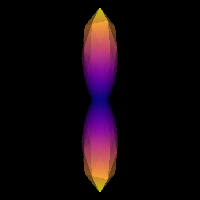## Contextual enhancement

### Crossing-preserving contextual enhancement

This demo presents an example of crossing-preserving contextual enhancement of FOD/ODF fields [Meesters2016], implementing the contextual PDE framework of [Portegies2015a] for processing HARDI data. The aim is to enhance the alignment of elongated structures in the data such that crossing/junctions are maintained while reducing noise and small incoherent structures. This is achieved via a hypo-elliptic 2nd order PDE in the domain of coupled positions and orientations $$\mathbb{R}^3 \rtimes S^2$$. This domain carries a non-flat geometrical differential structure that allows including a notion of alignment between neighboring points.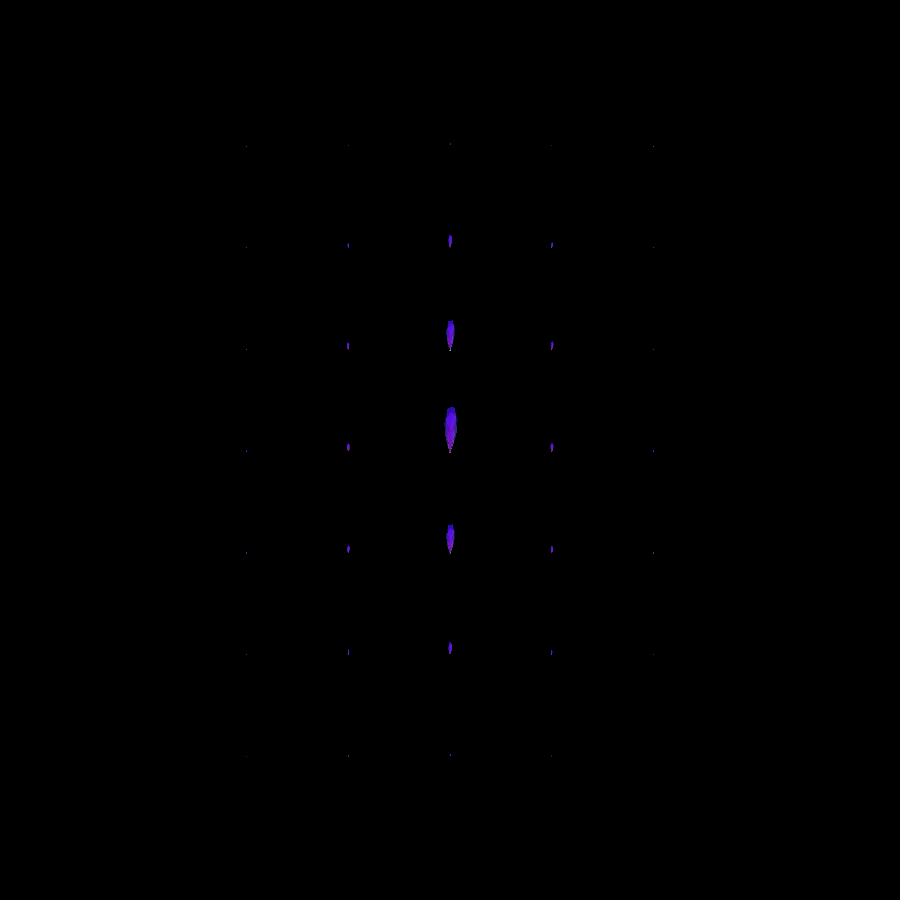### Fiber to bundle coherence measures

This demo presents the fiber to bundle coherence (FBC) quantitative measure of the alignment of each fiber with the surrounding fiber bundles [Meesters2016]. These measures are useful in ‘cleaning’ the results of tractography algorithms, since low FBCs indicate which fibers are isolated and poorly aligned with their neighbors, as shown in the figure below.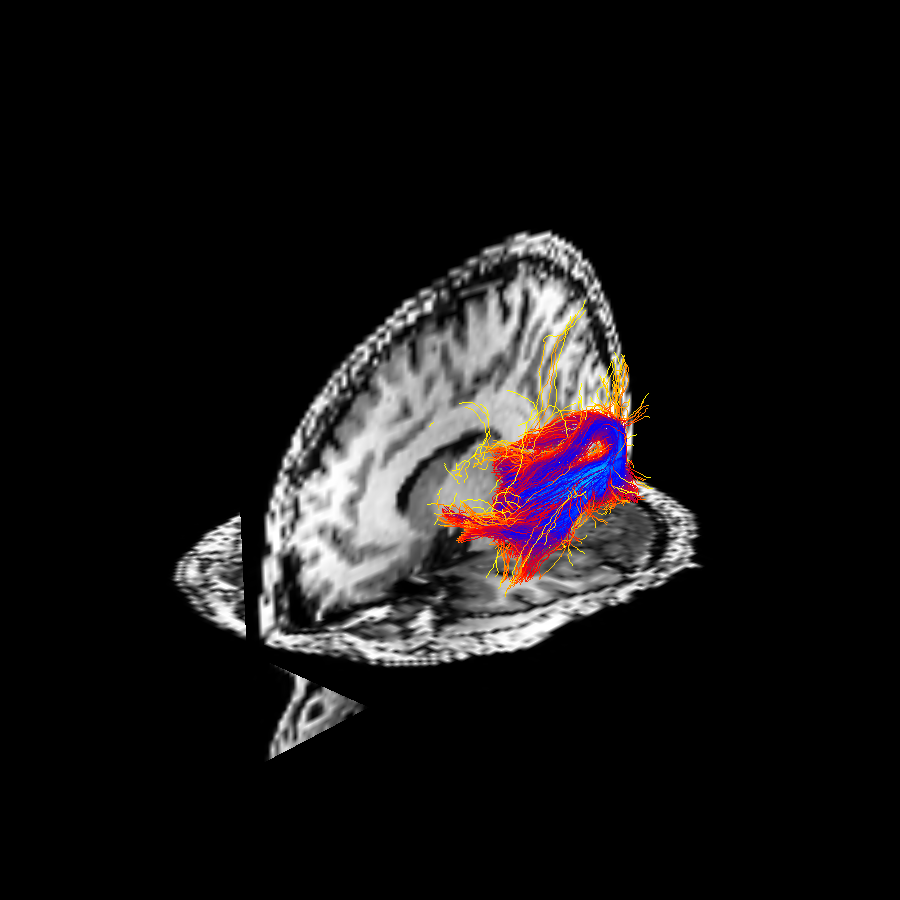## Fiber tracking

### Introduction to Basic Tracking

Local fiber tracking is an approach used to model white matter fibers by creating streamlines from local directional information. The idea is as follows: if the local directionality of a tract/pathway segment is known, one can integrate along those directions to build a complete representation of that structure. Local fiber tracking is widely used in the field of diffusion MRI because it is simple and robust.### An introduction to the Deterministic Maximum Direction Getter

Deterministic maximum direction getter is the deterministic version of the probabilistic direction getter. It can be used with the same local models and has the same parameters. Deterministic maximum fiber tracking follows the trajectory of the most probable pathway within the tracking constraint (e.g. max angle). In other words, it follows the direction with the highest probability from a distribution, as opposed to the probabilistic direction getter which draws the direction from the distribution. Therefore, the maximum deterministic direction getter is equivalent to the probabilistic direction getter returning always the maximum value of the distribution.

### An introduction to the Probabilistic Direction Getter

Probabilistic fiber tracking is a way of reconstructing white matter connections using diffusion MR imaging. Like deterministic fiber tracking, the probabilistic approach follows the trajectory of a possible pathway step by step starting at a seed, however, unlike deterministic tracking, the tracking direction at each point along the path is chosen at random from a distribution. The distribution at each point is different and depends on the observed diffusion data at that point. The distribution of tracking directions at each point can be represented as a probability mass function (PMF) if the possible tracking directions are restricted to discrete numbers of well distributed points on a sphere.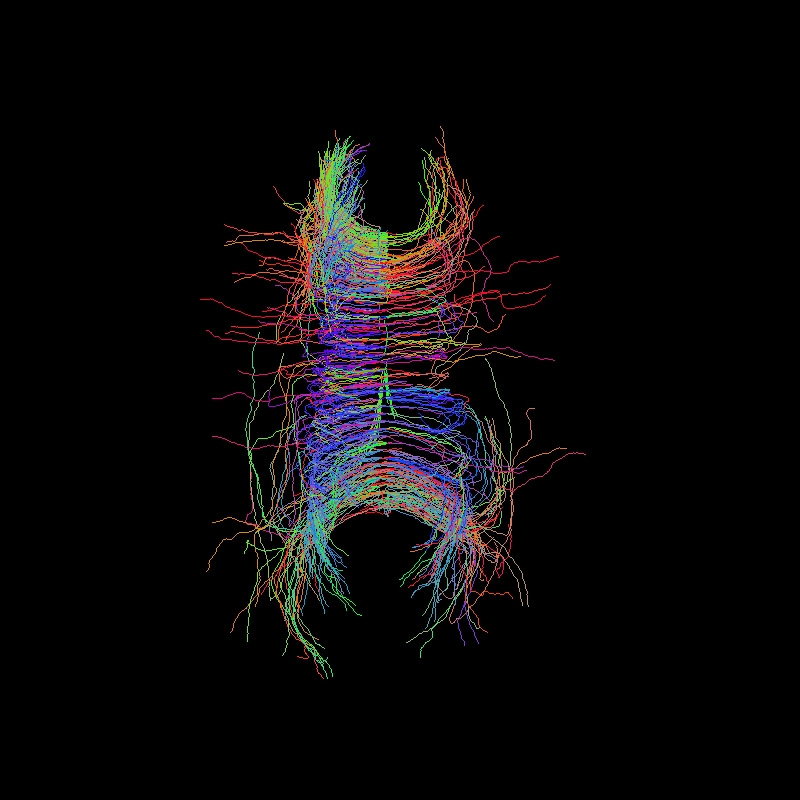### Bootstrap and Closest Peak Direction Getters Example

This example shows how choices in direction-getter impact fiber tracking results by demonstrating the bootstrap direction getter (a type of probabilistic tracking, as described in Berman et al. (2008) [Berman2008] a nd the closest peak direction getter (a type of deterministic tracking). (Amirbekian, PhD thesis, 2016)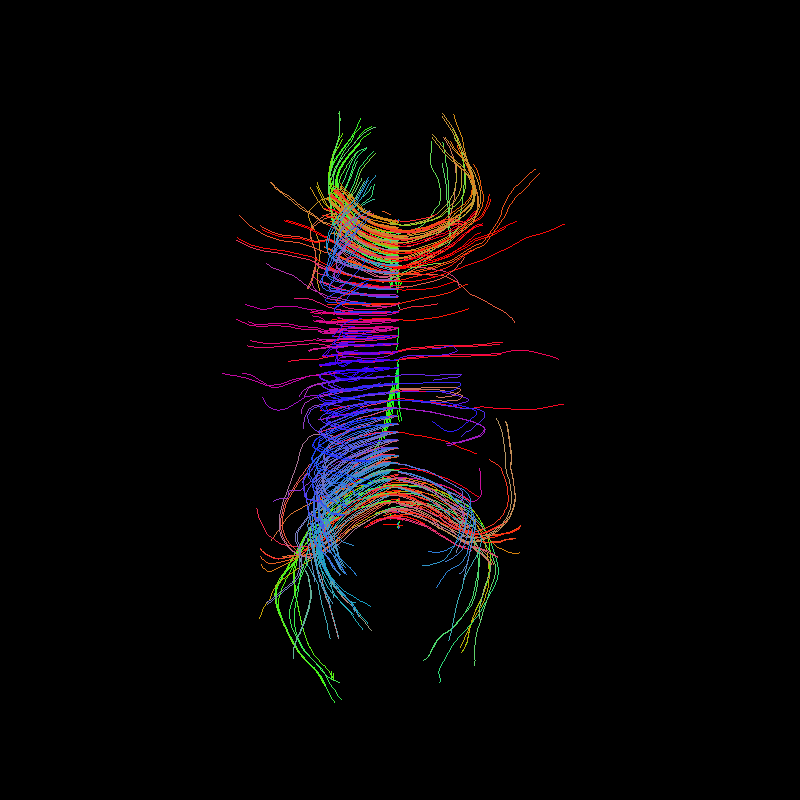### Using Various Stopping Criterion for Tractography

The stopping criterion determines if the tracking stops or continues at each tracking position. The tracking stops when it reaches an ending region (e.g. low FA, gray matter or corticospinal fluid regions) or exits the image boundaries. The tracking also stops if the direction getter has no direction to follow.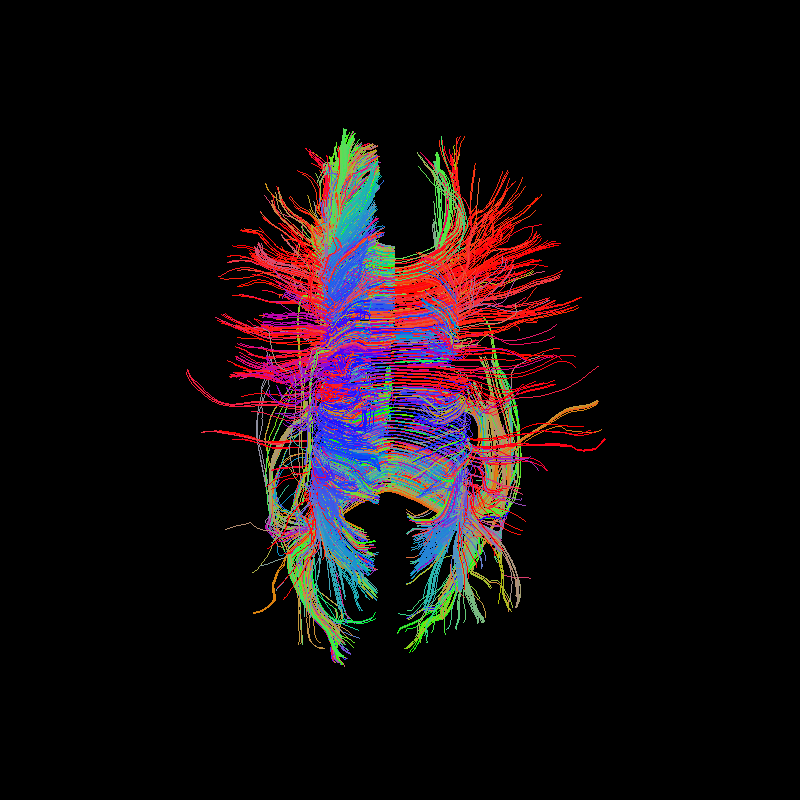### Particle Filtering Tractography

Particle Filtering Tractography (PFT) [Girard2014] uses tissue partial volume estimation (PVE) to reconstruct trajectories connecting the gray matter, and not incorrectly stopping in the white matter or in the corticospinal fluid. It relies on a stopping criterion that identifies the tissue where the streamline stopped. If the streamline correctly stopped in the gray matter, the trajectory is kept. If the streamline incorrectly stopped in the white matter or in the corticospinal fluid, PFT uses anatomical information to find an alternative streamline segment to extend the trajectory. When this segment is found, the tractography continues until the streamline correctly stops in the gray matter.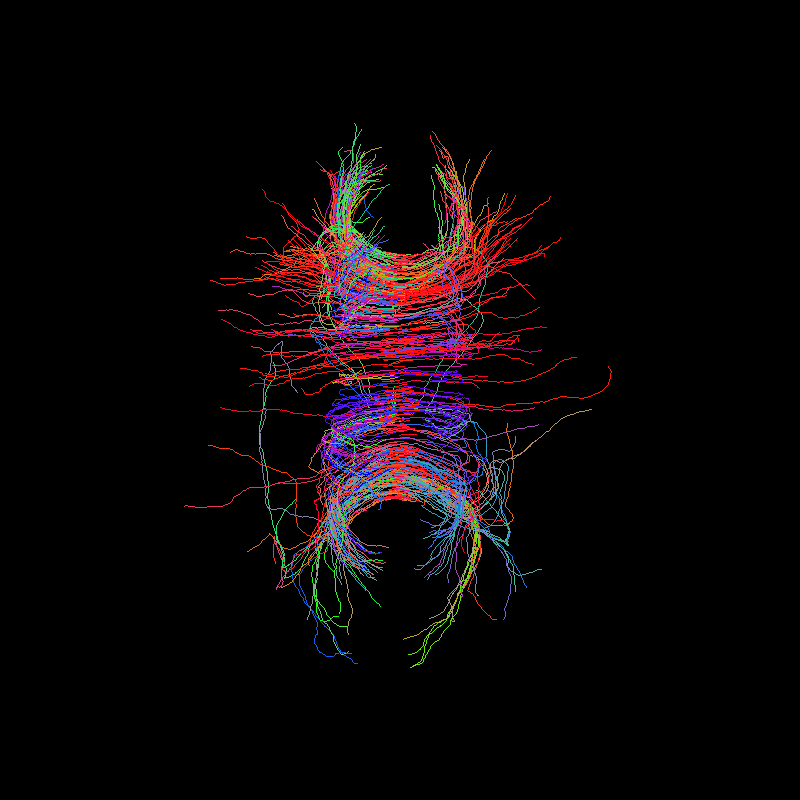### Tracking with the Sparse Fascicle Model

Tracking requires a per-voxel model. Here, the model is the Sparse Fascicle Model (SFM), described in [Rokem2015]. This model reconstructs the diffusion signal as a combination of the signals from different fascicles (see also sfm-reconst).

### Tracking with Robust Unbiased Model-BAsed Spherical Deconvolution (RUMBA-SD)

Here, we demonstrate fiber tracking using a probabilistic direction getter and RUMBA-SD, a model introduced in [CanalesRodriguez2015]. This model adapts Richardson-Lucy deconvolution by assuming Rician or Noncentral Chi noise instead of Gaussian, which more accurately reflects the noise from MRI scanners (see also Reconstruction with Robust and Unbiased Model-BAsed Spherical Deconvolution). This tracking tutorial is an extension on An introduction to the Probabilistic Direction Getter.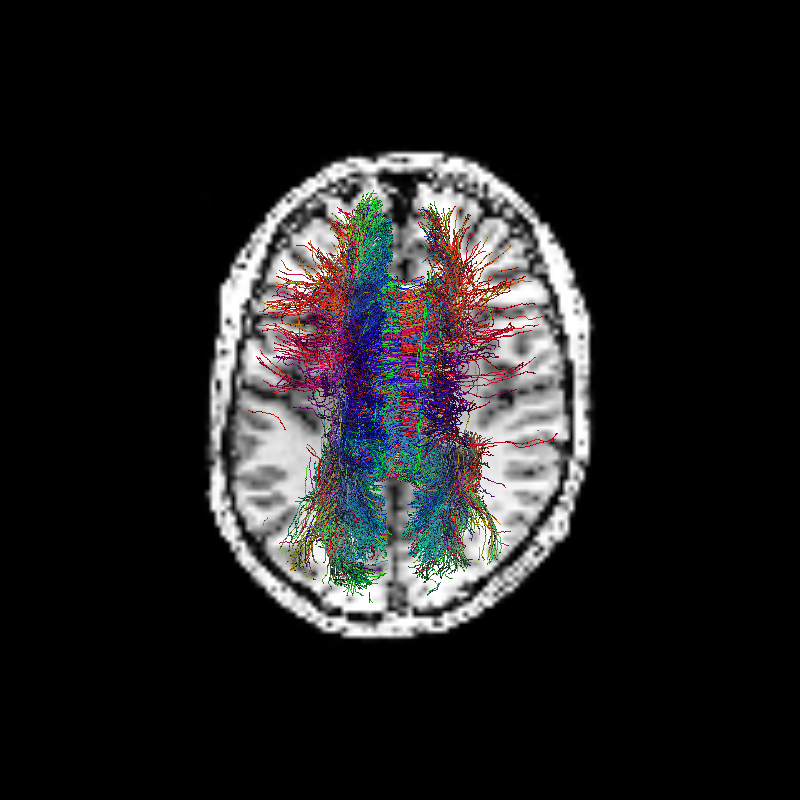### Linear fascicle evaluation (LiFE)

Evaluating the results of tractography algorithms is one of the biggest challenges for diffusion MRI. One proposal for evaluation of tractography results is to use a forward model that predicts the signal from each of a set of streamlines, and then fit a linear model to these simultaneous predictions [Pestilli2014].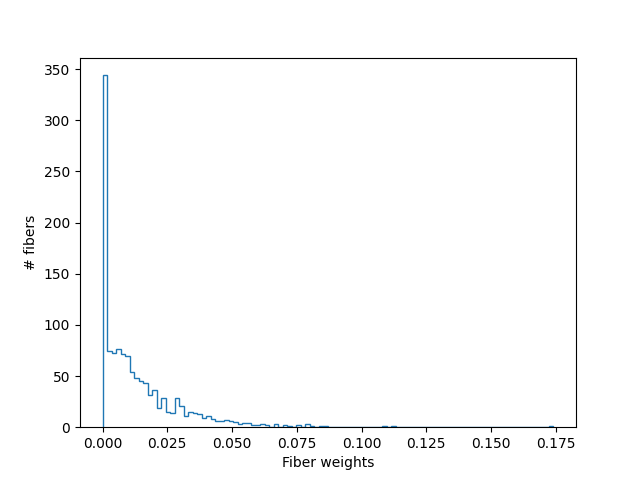### Surface seeding for tractography

Surface seeding is a way to generate initial position for tractography from cortical surfaces position [Stonge2018].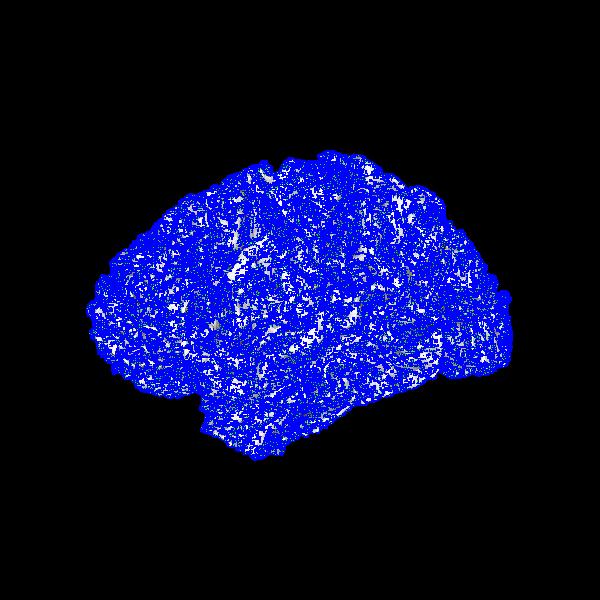## Streamline analysis and connectivity

### Connectivity Matrices, ROI Intersections and Density Maps

This example is meant to be an introduction to some of the streamline tools available in DIPY. Some of the functions covered in this example are target, connectivity_matrix and density_map. target allows one to filter streamlines that either pass through or do not pass through some region of the brain, connectivity_matrix groups and counts streamlines based on where in the brain they begin and end, and finally, density map counts the number of streamlines that pass through every voxel of some image.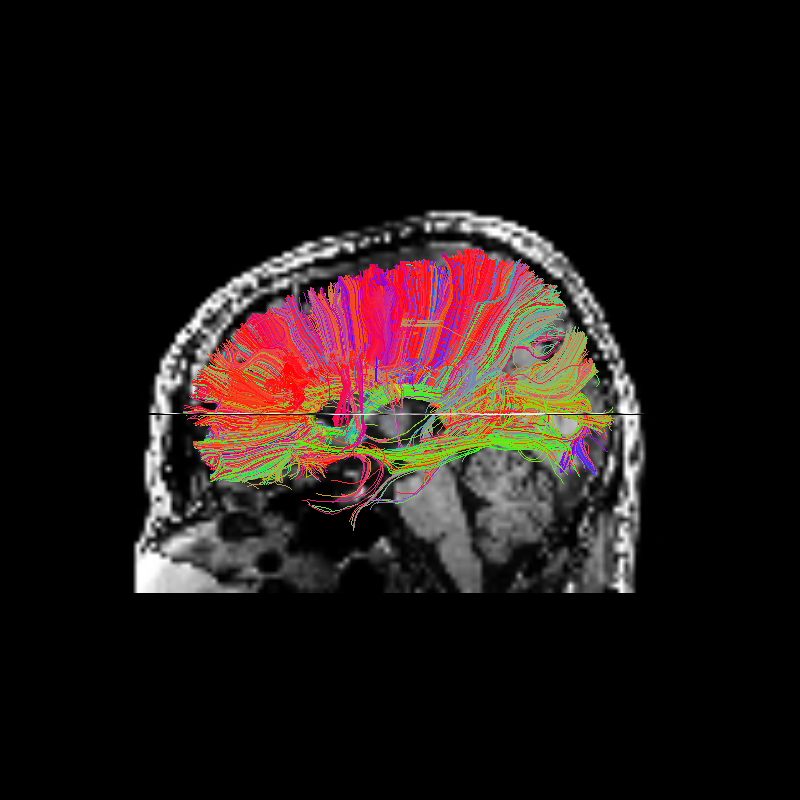### Streamline length and size reduction

This example shows how to calculate the lengths of a set of streamlines and also how to compress the streamlines without considerably reducing their lengths or overall shape.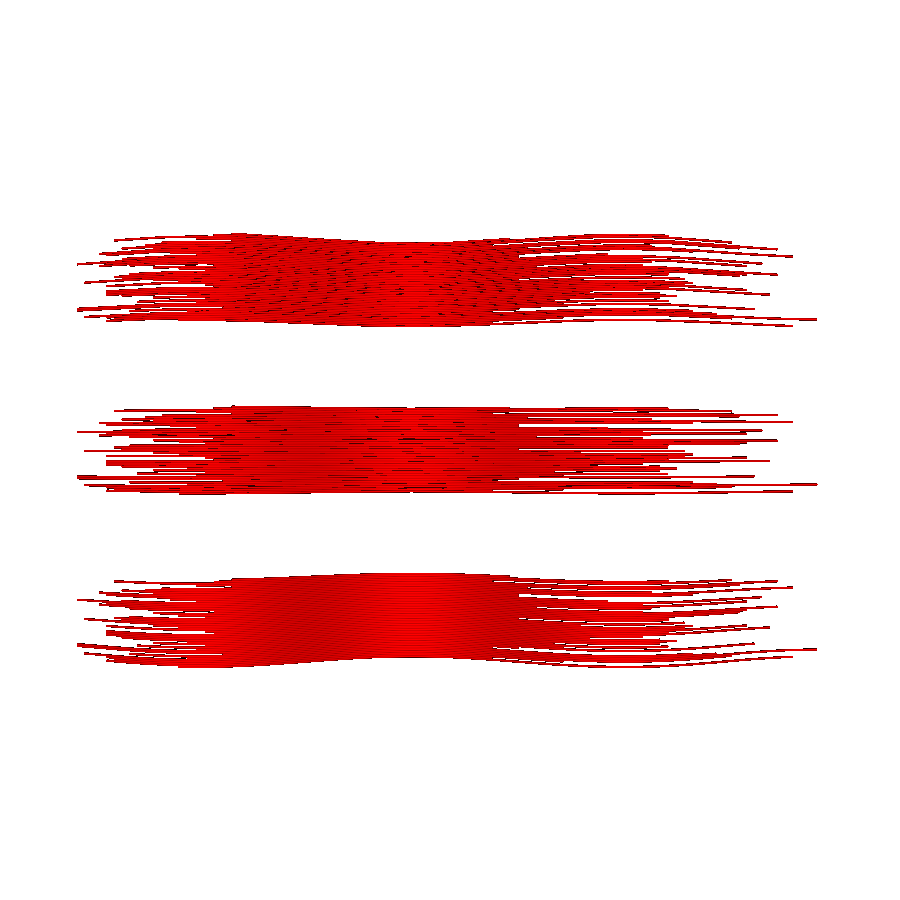### Calculation of Outliers with Cluster Confidence Index

This is an outlier scoring method that compares the pathways of each streamline in a bundle (pairwise) and scores each streamline by how many other streamlines have similar pathways. The details can be found in [Jordan_2018_plm].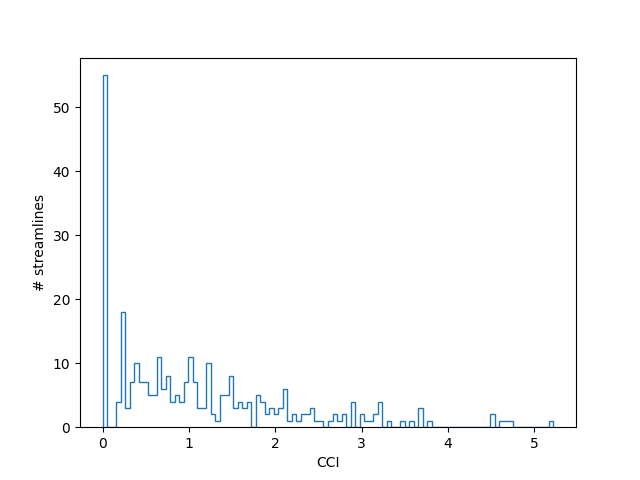### Calculate Path Length Map

We show how to calculate a Path Length Map for Anisotropic Radiation Therapy Contours given a set of streamlines and a region of interest (ROI). The Path Length Map is a volume in which each voxel’s value is the shortest distance along a streamline to a given region of interest (ROI). This map can be used to anisotropically modify radiation therapy treatment contours based on a tractography model of the local white matter anatomy, as described in [Jordan_2018_plm], by executing this tutorial with the gross tumor volume (GTV) as the ROI.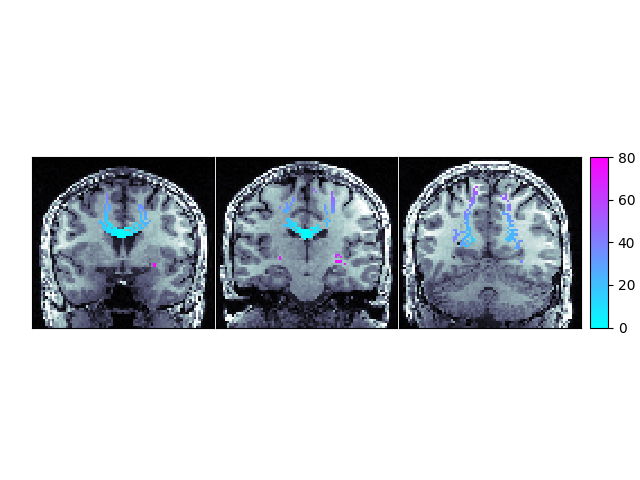### Extracting AFQ tract profiles from segmented bundles

In this example, we will extract the values of a statistic from a volume, using the coordinates along the length of a bundle. These are called tract profiles

### BUAN Bundle Assignment Maps Creation

This example explains how we can use BUAN [Chandio2020] to create assignment maps on a bundle. Divide bundle into N smaller segments.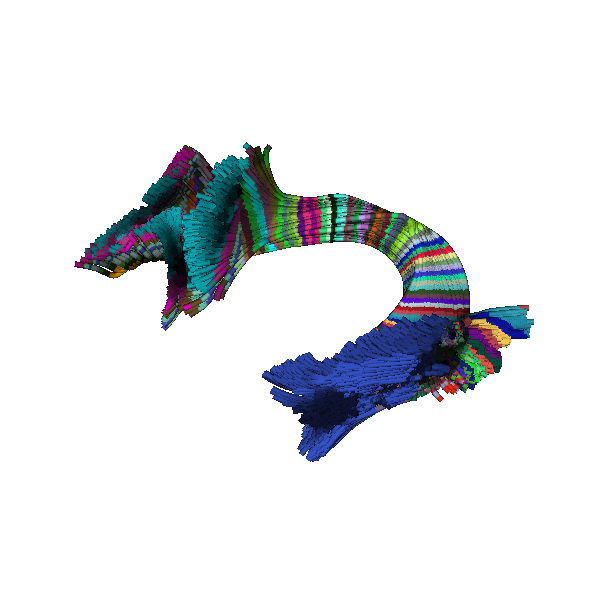### BUAN Bundle Shape Similarity Score

This example explains how we can use BUAN [Chandio2020] to calculate shape similarity between two given bundles. Where, shape similarity score of 1 means two bundles are extremely close in shape and 0 implies no shape similarity whatsoever.

## Registration

### Affine Registration in 3D

This example explains how to compute an affine transformation to register two 3D volumes by maximization of their Mutual Information [Mattes03]. The optimization strategy is similar to that implemented in ANTS [Avants11].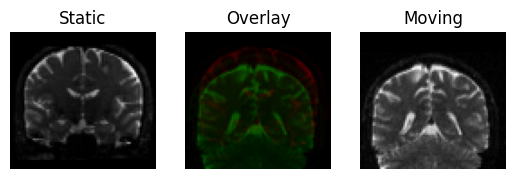This example explains how to compute a transformation to register two 3D volumes by maximization of their Mutual Information [Mattes03]. The optimization strategy is similar to that implemented in ANTS [Avants11].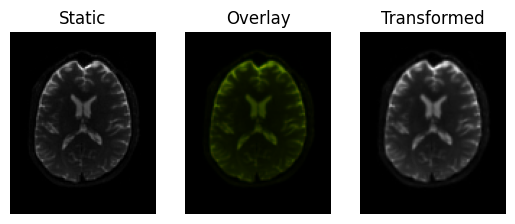### Symmetric Diffeomorphic Registration in 2D

This example explains how to register 2D images using the Symmetric Normalization (SyN) algorithm proposed by Avants et al. [Avants09] (also implemented in the ANTs software [Avants11])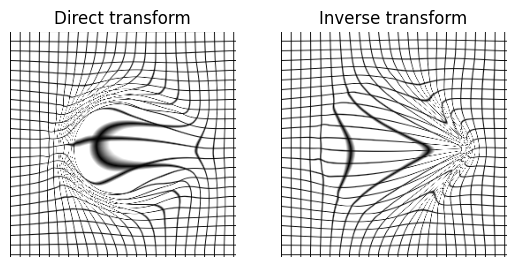### Symmetric Diffeomorphic Registration in 3D

This example explains how to register 3D volumes using the Symmetric Normalization (SyN) algorithm proposed by Avants et al. [Avants09] (also implemented in the ANTs software [Avants11])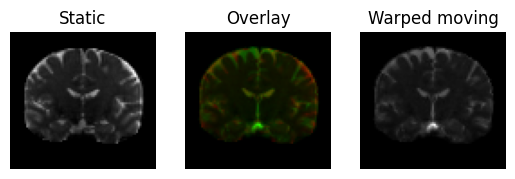### Diffeomorphic Registration with binary and fuzzy images

This example demonstrates registration of a binary and a fuzzy image. This could be seen as aligning a fuzzy (sensed) image to a binary (e.g., template) image.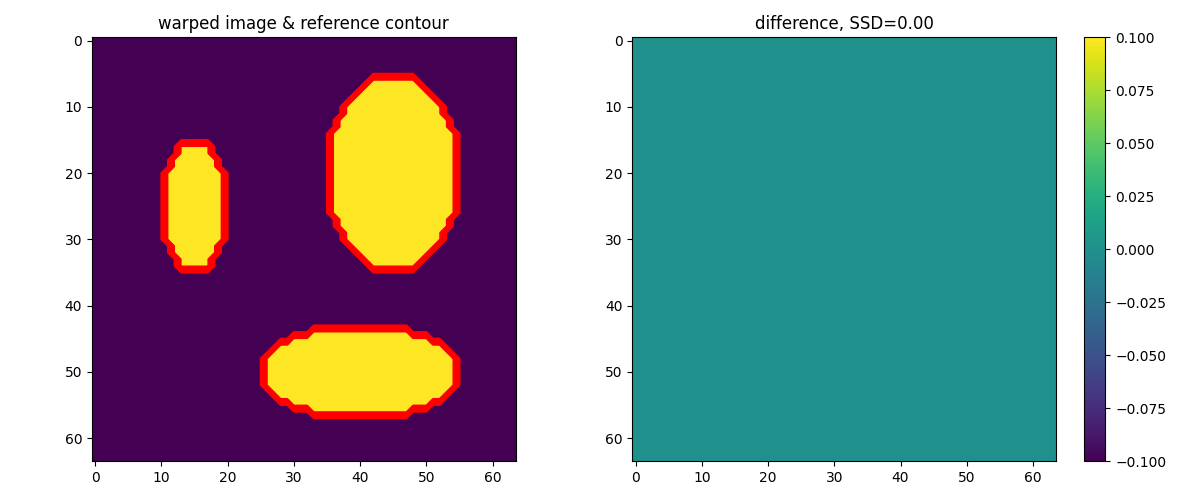### Direct Bundle Registration

This example explains how you can register two bundles from two different subjects directly in the space of streamlines [Garyfallidis15], [Garyfallidis14].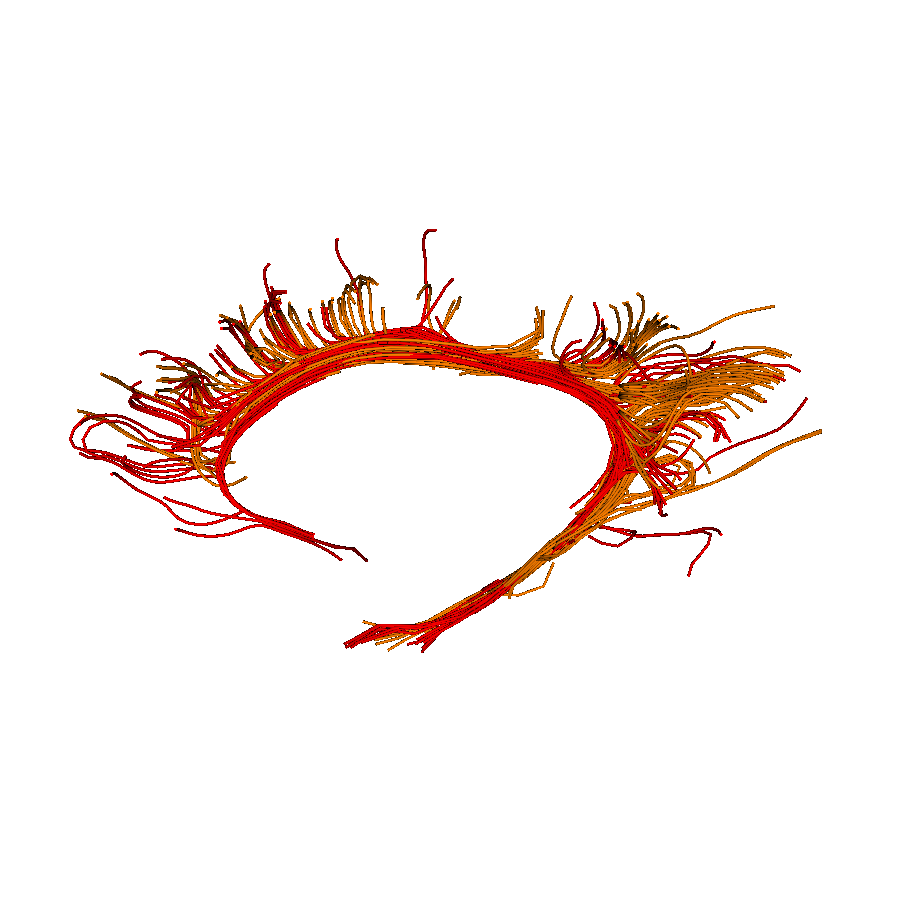### Groupwise Bundle Registration

This example explains how to coregister a set of bundles to a common space that is not biased by any specific bundle. This is useful when we want to align all the bundles but do not have a target reference space defined by an atlas.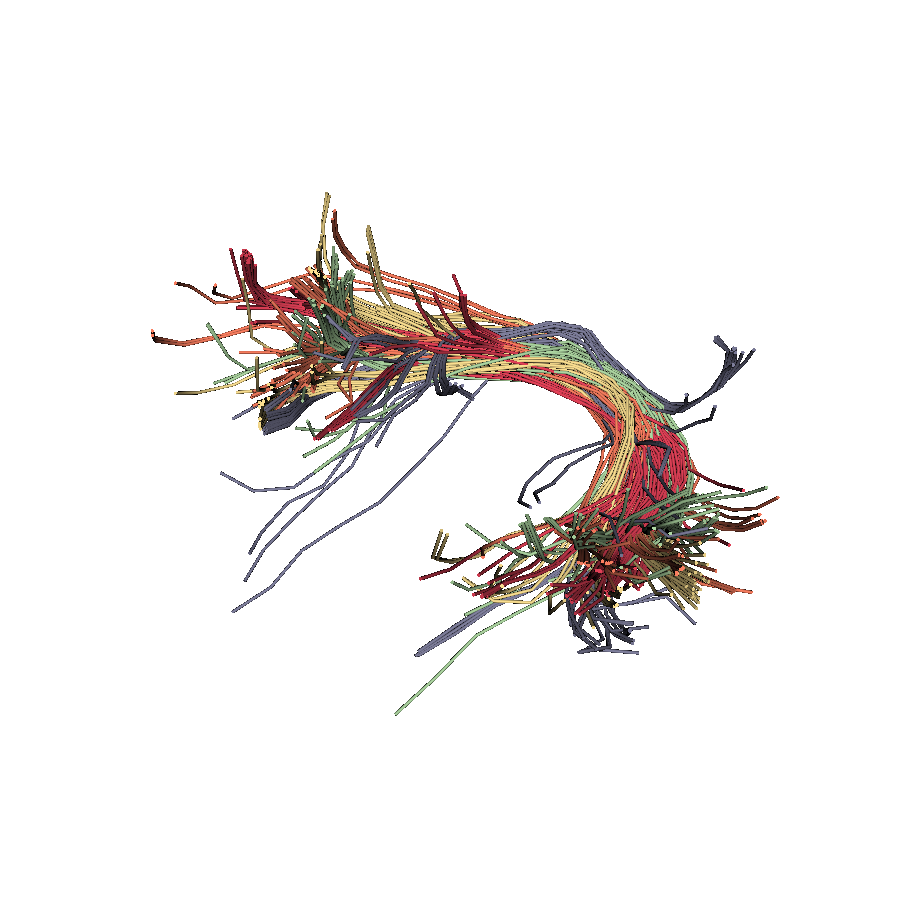### Applying image-based deformations to streamlines

This example shows how to register streamlines into a template space by applying non-rigid deformations.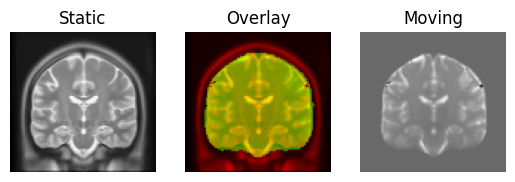## Segmentation

### Tractography Clustering with QuickBundles

This example explains how we can use QuickBundles [Garyfallidis12] to simplify/cluster streamlines.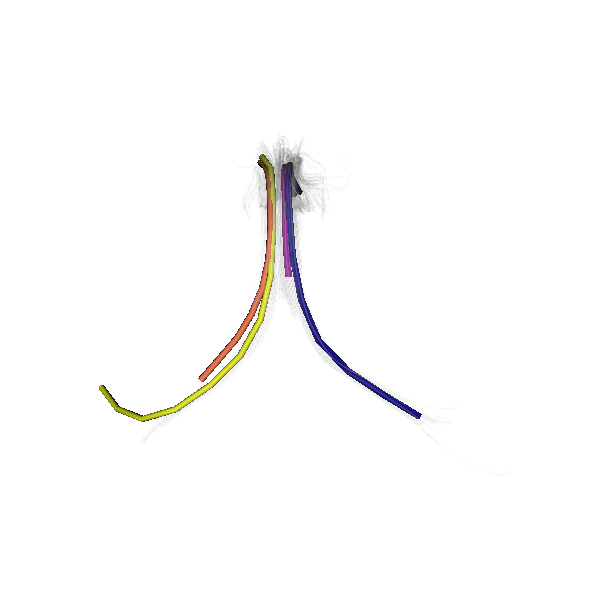### Enhancing QuickBundles with different metrics and features

QuickBundles [Garyfallidis12] is a flexible algorithm that requires only a distance metric and an adjacency threshold to perform clustering. There is a wide variety of metrics that could be used to cluster streamlines.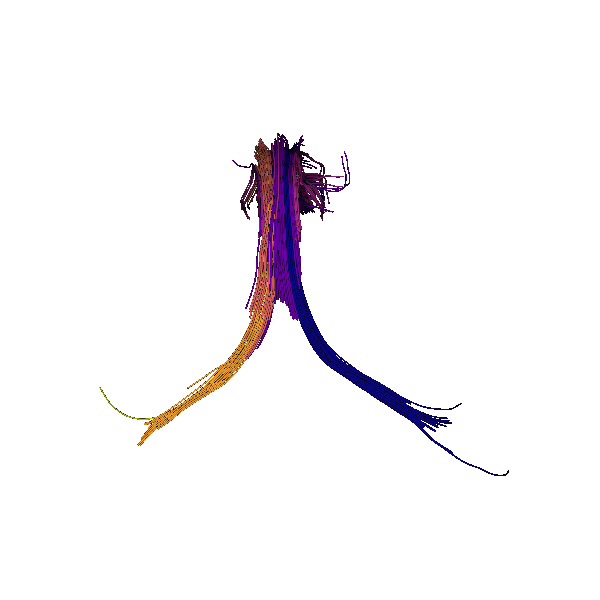### Tractography Clustering - Available Features

This page lists available features that can be used by the tractography clustering framework. For every feature a brief description is provided explaining: what it does, when it’s useful and how to use it. If you are not familiar with the tractography clustering framework, read the Clustering framework first.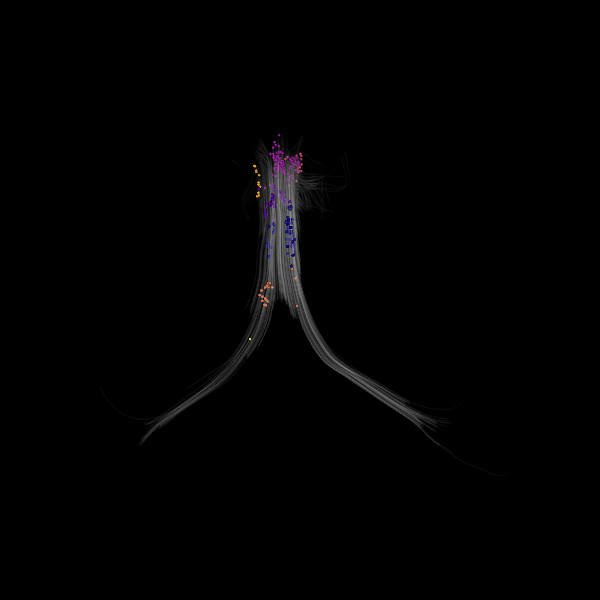### Tractography Clustering - Available Metrics

This page lists available metrics that can be used by the tractography clustering framework. For every metric a brief description is provided explaining: what it does, when it’s useful and how to use it. If you are not familiar with the tractography clustering framework, check this tutorial Clustering framework.

### Brain segmentation with median_otsu

We show how to extract brain information and mask from a b0 image using DIPY’s segment.mask module.

### Tissue Classification of a T1-weighted Structural Image

This example explains how to segment a T1-weighted structural image by using Bayesian formulation. The observation model (likelihood term) is defined as a Gaussian distribution and a Markov Random Field (MRF) is used to model the a priori probability of context-dependent patterns of different tissue types of the brain. Expectation Maximization and Iterated Conditional Modes are used to find the optimal solution. Similar algorithms have been proposed by Zhang et al. [Zhang2001] and Avants et al. [Avants2011] available in FAST-FSL and ANTS-atropos, respectively.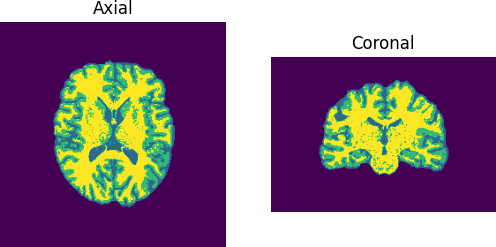### Automatic Fiber Bundle Extraction with RecoBundles

This example explains how we can use RecoBundles [Garyfallidis17] to extract bundles from tractograms.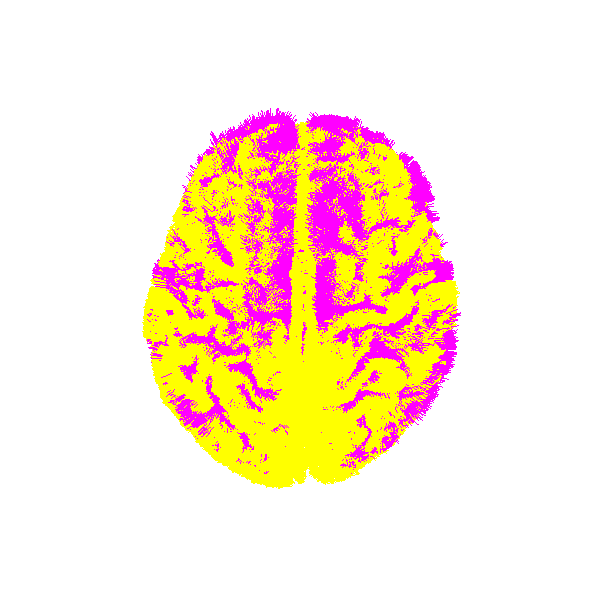## Simulations

### MultiTensor Simulation

In this example we show how someone can simulate the signal and the ODF of a single voxel using a MultiTensor.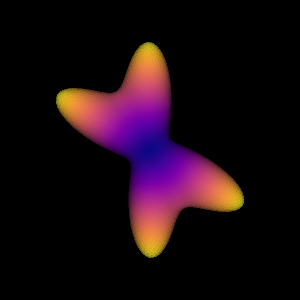### DSI Deconvolution vs DSI

An alternative method to DSI is the method proposed by [Canales10] which is called DSI with Deconvolution. This algorithm is using Lucy-Richardson deconvolution in the diffusion propagator with the goal to create sharper ODFs with higher angular resolution.

### DKI MultiTensor Simulation

In this example we show how to simulate the Diffusion Kurtosis Imaging (DKI) data of a single voxel. DKI captures information about the non-Gaussian properties of water diffusion which is a consequence of the existence of tissue barriers and compartments. In these simulations compartmental heterogeneity is taken into account by modeling different compartments for the intra- and extra-cellular media of two populations of fibers. These simulations are performed according to [RNH2015].

## Multiprocessing

### Parallel reconstruction using CSD

This example shows how to use parallelism (multiprocessing) using peaks_from_model in order to speedup the signal reconstruction process. For this example will we use the same initial steps as we used in Reconstruction with Constrained Spherical Deconvolution.

### Parallel reconstruction using Q-Ball

We show an example of parallel reconstruction using a Q-Ball Constant Solid Angle model (see Aganj et al. (MRM 2010)) and peaks_from_model.

## File Formats

DIPY_ can read and write many different file formats. In this example we give a short introduction on how to use it for loading or saving streamlines. The new stateful tractogram class was made to reduce errors caused by spatial transformation and complex file format convention.

## Visualization

In DIPY we created a thin interface to access many of the capabilities available in the FURY 3D visualization library but tailored to the needs of structural and diffusion imaging.

### Simple volume slicing

Here we present an example for visualizing slices from 3D images.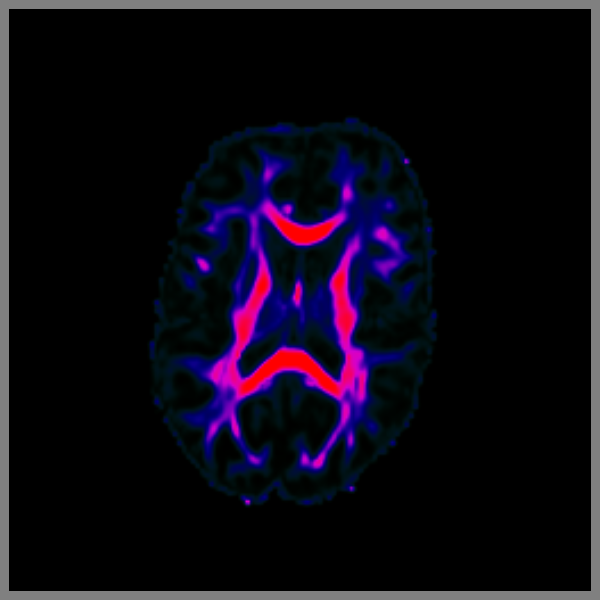### Visualize bundles and metrics on bundles

First, let’s download some available datasets. Here we are using a dataset which provides metrics and bundles.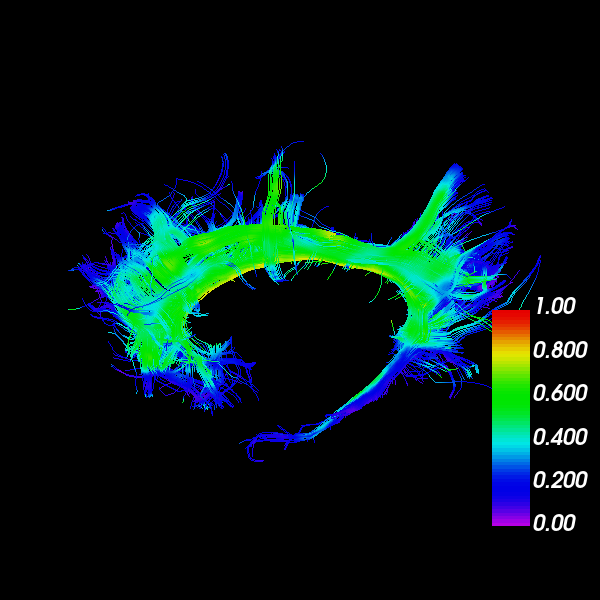### Visualization of ROI Surface Rendered with Streamlines

Here is a simple tutorial following the probabilistic CSA Tracking Example in which we generate a dataset of streamlines from a corpus callosum ROI, and then display them with the seed ROI rendered in 3D with 50% transparency.

## Workflows

### Creating a new workflow.

A workflow is a series of DIPY operations with fixed inputs and outputs that is callable via command line or another interface.

### Creating a new combined workflow

A CombinedWorkflow is a series of DIPY workflows organized together in a way that the output of a workflow serves as input for the next one.